# Python中的self用法详解

class是面向对象的设计思想，instance（也即是 object，对象）是根据 class 创建的。

• -> 而需要访问实例的变量和调用实例的函数，当然需要对应的实例Instance对象本身。
• -> 而Python中就规定好了，函数的第一个参数，就必须是实例对象本身，并且建议，约定俗成，把其名字写为self。
• -> 所以，我们需要self（需要用到self）。

class 后面紧跟类名，即 Person，类名通常大写字母开头，紧接着是(object),表示该类是从哪个类继承下来的，通常，如果没有合适的 继承类，就使用 object 类，这是所有类最终都会继承的类。

```class Person（object）:
pass```

```class Person(object):
pass
student = Person()  # 创建类的实例化
print(student)
print(Person)```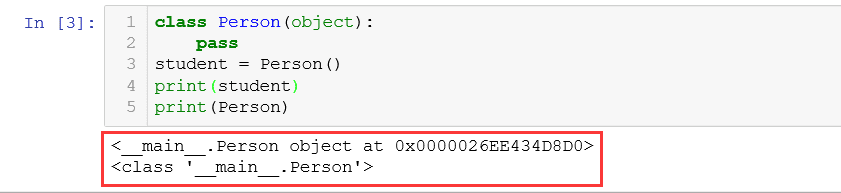```class Person(object):
pass
student = Person()
# print(student)
# print(Person)
student.name = "Gavin"   # 为实例变量
student 绑定 name 属性  类似于 赋值 操作
student.score = 100    # 为 其绑定
score 属性
print(student.name)p
rint(student.score)```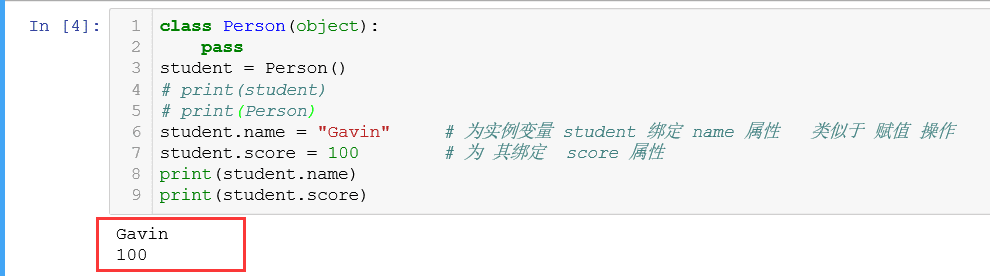```class Person(object):
def __init__(self,name,score):
self.name = name
self.score = score
student = Person('Gavin',100)  # 传入 __init__ 方法中需要的参数
print(student.name)
print(student.score)```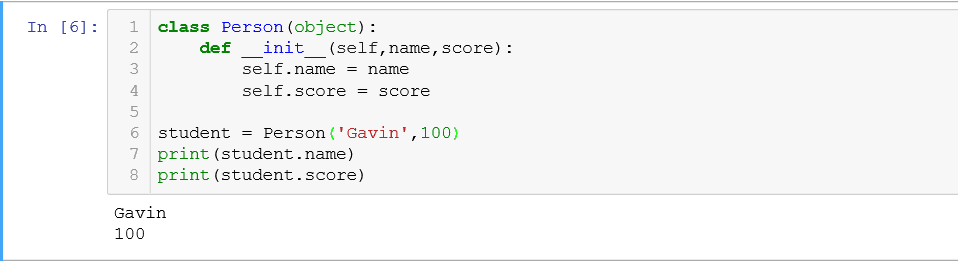```class Person(object):
def __init__(self,name,score):
self.name = name
self.score = score
student = Person()   # 此处应该有参数传入，却没有传
print(student.name)
print(student.score)```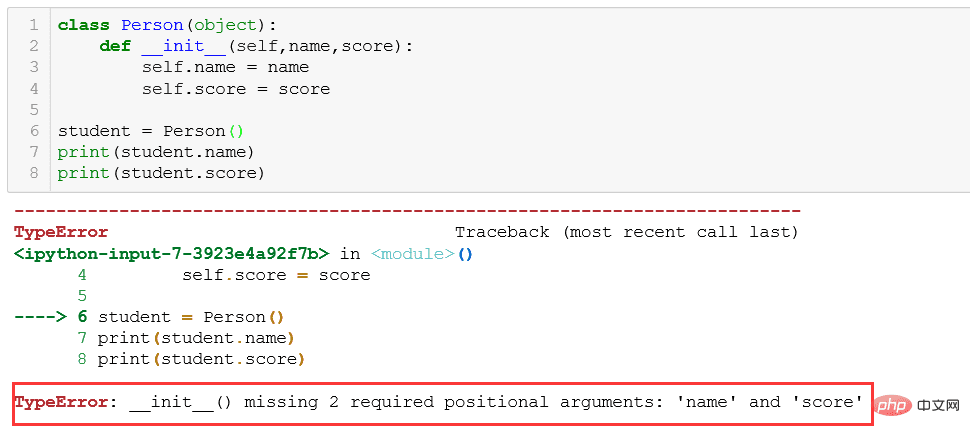1、__init__ 方法的第一个参数永远是 self ，表示创建的实例本身，因此，在 __init__ 方法的内部，就可以把各种属性绑定到 self，因为 self 就指向创建的实例本身。

2、使用了 __init__ 方法，在创建实例的时候就不能传入 空的参数了，必须传入与 __init__ 方法匹配的参数，但是 self 不需要传，python解释器会自己把实例变量传进去。

```class Person(object):
def __init__(self,x,y):
self.x = x
self.y = y
sum = self.x + self.y
return sum
def square(self):
squr = pow(self.x,2)+pow(self.y,2)
return squr
return c
print(student.square())
print('--------- 我是可爱的分割线-----------')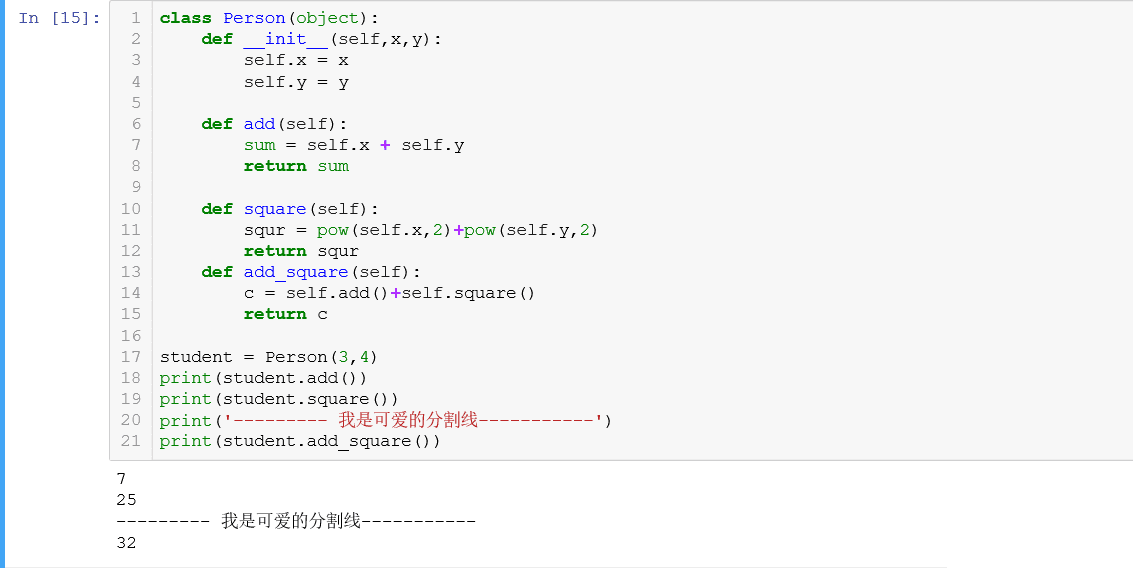1、第一个参数永远是 self ，并且调用时不用传递该参数

2、在类中函数相互调用要加 self ，如上例中： c = self.add()+self.square(), 不加 self ，会报错： 函数未定义，看下图：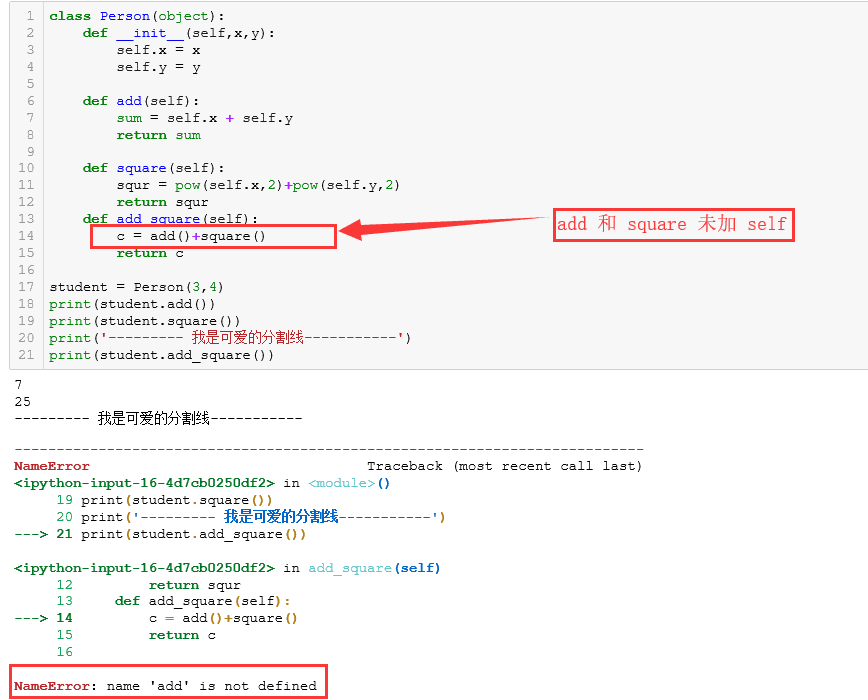```class Person(object):
def __init__(self,x,y):
self.x = x
self.y = y
def add(self,z=16):     # 设置 默认变量 z =16,这只是个普通的局部变量，非实例变量，实例变量需要
self.z = z,这样定义
sum = self.x + self.y + z
return sum
def square(self):
squr = pow(self.x,2)+pow(self.y,2)
return squr
return c
student = Person(3,4)
print(student.square())
print('--------- 我是可爱的分割线-----------')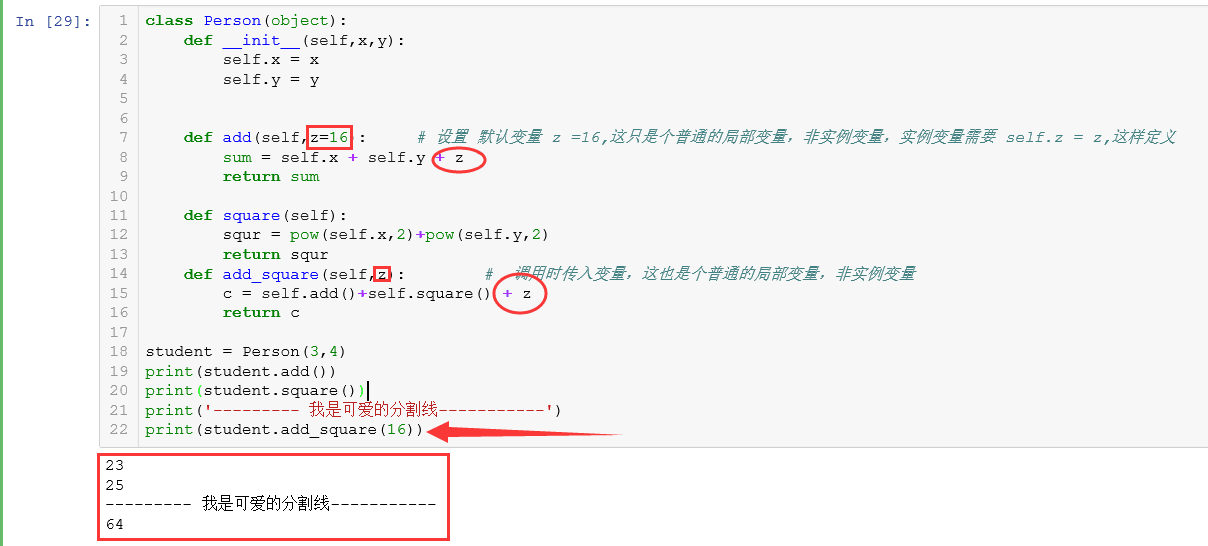```class Person(object):
def __init__(self,x,y):
self.x = x
self.y = y
def add(self,z=16):   # 设置 z 为实例变量，即
self.z = z, z 是 class 的一个成员了，而非普通局部变量
self.z = z
sum = self.x + self.y + z # z虽然已被实例化，但是依然可以当作 普通变量来用    r
eturn sum
def square(self):
squr = pow(self.x,2)+pow(self.y,2)
return squr
c = self.add()+self.square() + self.z # 调用实例变量 z
return c
student = Person(3,4)
print(student.square())
print('--------- 我是可爱的分割线-----------')
print(student.z)     # 函数add 中的 z 被实例化以后，就可以利用实例化的方法访问它```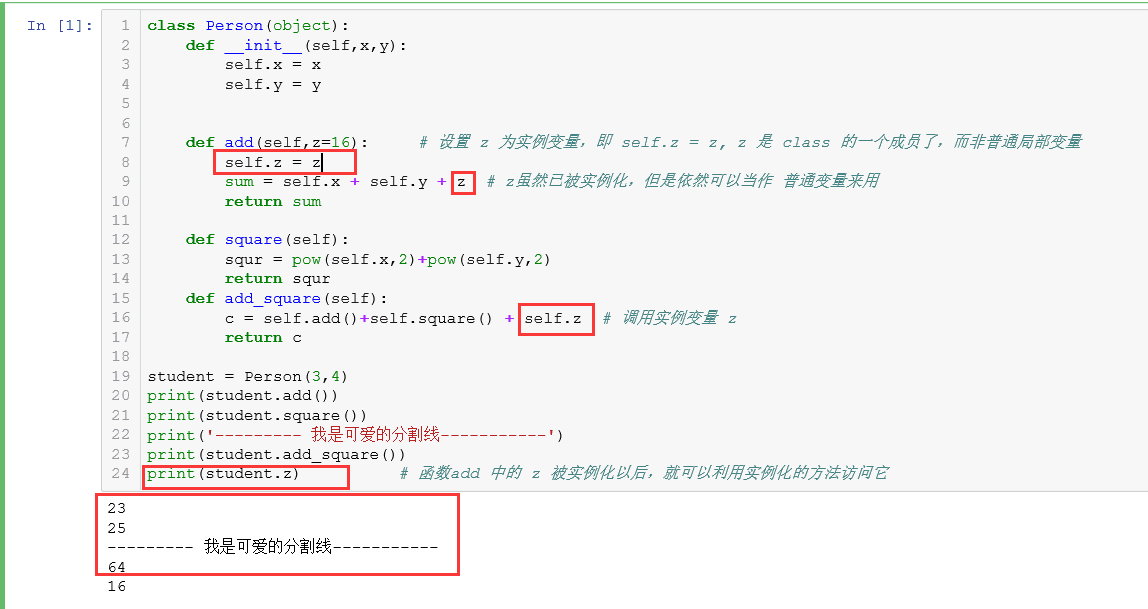```class Box(object):

def __init__(self, boxname, size, color):

self.boxname = boxname

self.size = size

self.color = color # self就是用于存储对象属性的集合，就算没有属性self也是必备的

def open(self, myself):

print('-->用自己的myself，打开那个%s,%s的%s' % (myself.color, myself.size, myself.boxname))

print('-->用类自己的self，打开那个%s,%s的%s' % (self.color, self.size, self.boxname))

def close(self):

print('-->关闭%s，谢谢' % self.boxname)

b = Box('魔盒', '14m', '红色')

b.close()

b.open(b) # 本来就会自动传一个self，现在传入b，就会让open多得到一个实例对象本身，print看看是什么。

print(b.__dict__) # 这里返回的就是self本身，self存储属性，没有动作。```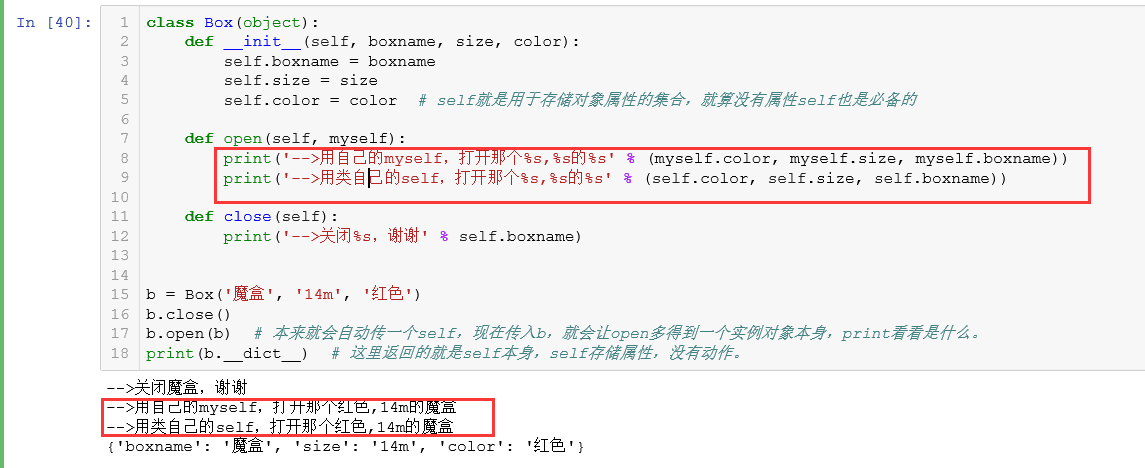self代表类的实例，而非类；self 就是 对象/实例 属性集合

Box 是个类-----》self 实例化------》 b对象/ 实例

class 抽象体------》实例化------》对象/实例，含有属性：{'boxname':'魔盒', ‘size'：‘14m', 'color':'red'}，即 self

self 看似是整个对象，实际上清楚地描述了类就是产生对象的过程，描述了 self 就是得到了 对象，所以 self 内的键值可以直接使用

1、描述对象的属性；2、对象的方法

```class Box(object):

def myInit(mySelf, boxname, size, color):

mySelf.boxname = boxname

mySelf.size = size

mySelf.color = color # 自己写一个初始化函数，一样奏效,甚至不用self命名。其它函数当中用标准self

return mySelf # 返回给实例化过程一个对象！神奇！并且含有对象属性/字典

# def __init__(self, boxname, size, color):

#   self.boxname = boxname

#   self.size = size

#   self.color = color #注释掉原来标准的初始化

def open(self, myself):

print(self)

print('-->用自己的myself，打开那个%s,%s的%s' % (myself.color, myself.size, myself.boxname))

print('-->用类自己的self，打开那个%s,%s的%s' % (myself.color, myself.size, myself.boxname))

def close(self):

print('-->关闭%s，谢谢' % self.boxname)

# 经过改造，运行结果和标准初始化没区别

b = Box().myInit('魔盒', '14m', '红色')

# b = Box('魔盒', '14m', '红色')#注释掉原来标准的初始化方法

b.close()

b.open(b) # 本来就会自动传一个self，现在传入b，就会让open多得到一个实例对象本身，print看看是什么。

print(b.__dict__) # 这里返回的就是self本身，self存储属性，没有动作。```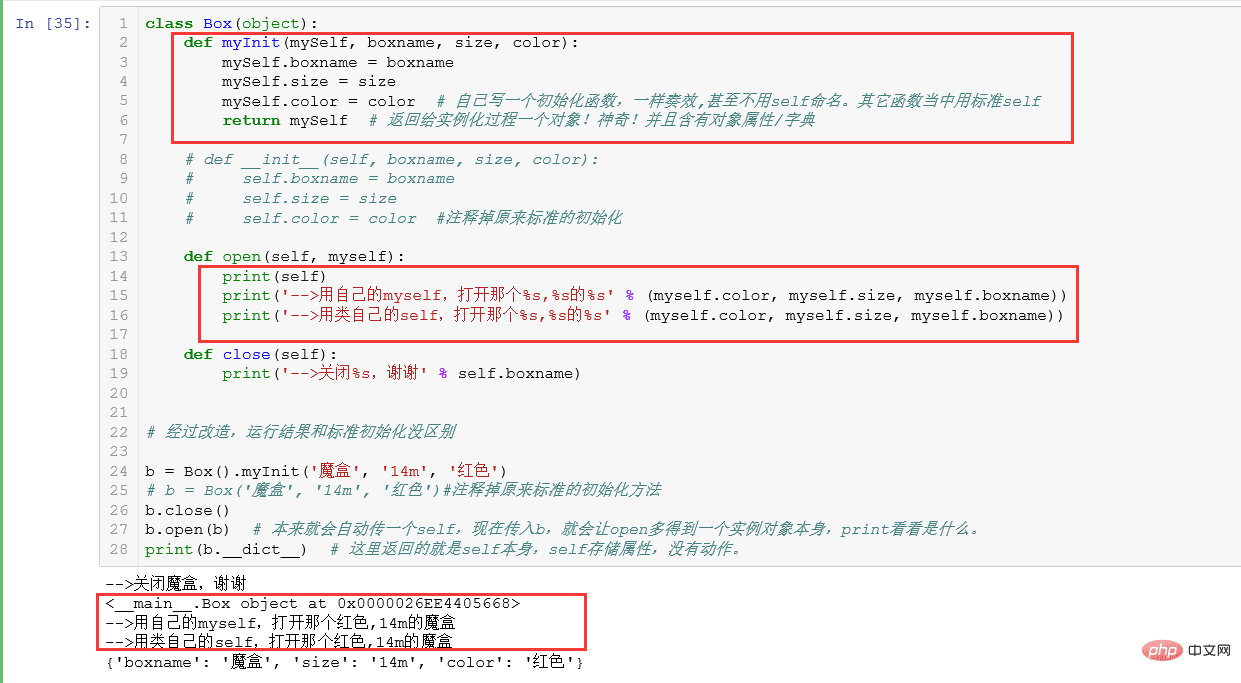1、定义属性 ； 2、调用方法

1、得到属性 ； 2、执行方法

```class Box(object):

def myInit(mySelf, boxname, size, color):

print(mySelf.__dict__)#显示为{}空字典

mySelf.boxname = boxname

mySelf.__dict__['aa'] = 'w'#甚至可以像字典一样操作

mySelf.size = size

mySelf.color = color # 自己写一个初始化函数，一样奏效,甚至不用self命名。其它函数当中用标准self

return mySelf # 返回给实例化过程一个对象！神奇！并且含有对象属性/字典

# def __init__(self, boxname, size, color):

#   self.boxname = boxname

#   self.size = size

#   self.color = color #注释掉原来标准的初始化

def open(self, myself):

print(self)

print('-->用自己的myself，打开那个%s,%s的%s' % (myself.color, myself.size, myself.boxname))

print('-->用类自己的self，打开那个%s,%s的%s' % (myself.color, myself.size, myself.boxname))

def close(self):

print('-->关闭%s，谢谢' % self.boxname)

# 经过改造，运行结果和标准初始化没区别

b = Box().myInit('魔盒', '14m', '红色')

# b = Box('魔盒', '14m', '红色')#注释掉原来标准的初始化方法

b.close()

b.open(b) # 本来就会自动传一个self，现在传入b，就会让open多得到一个实例对象本身，print看看是什么。

print(b.__dict__) # 这里返回的就是self本身，self存储属性，没有动作。```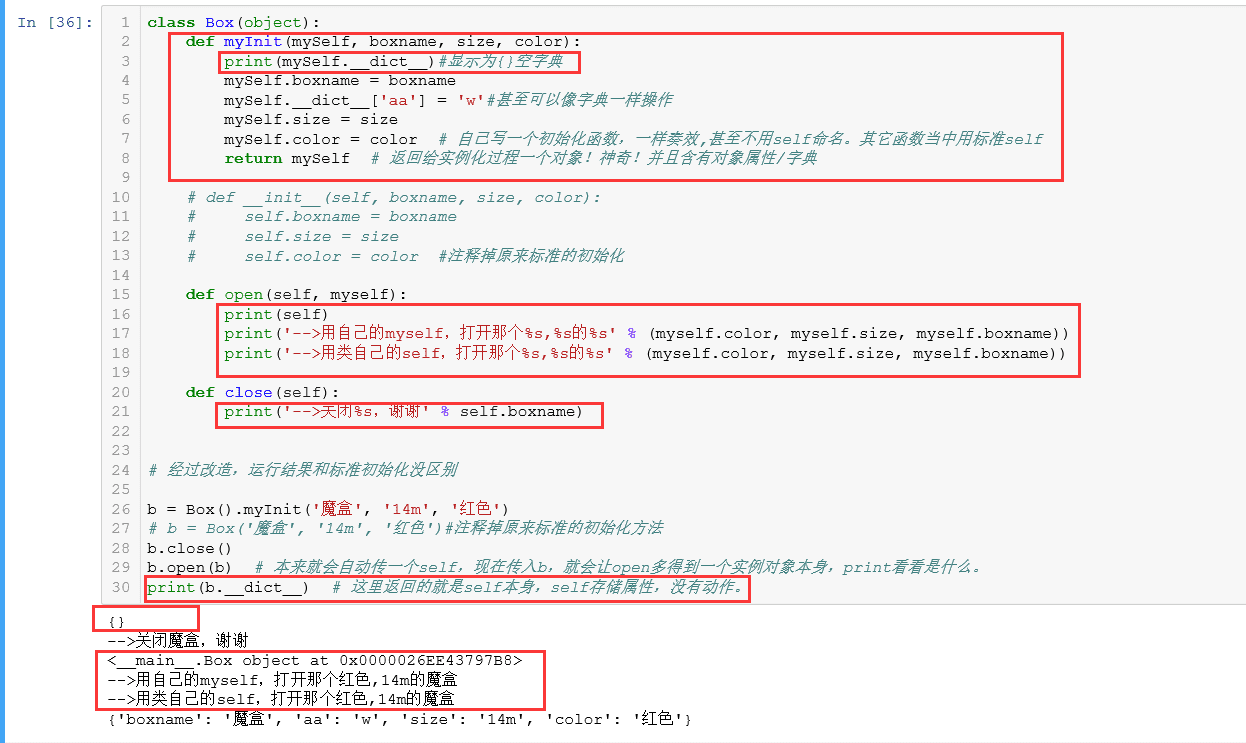self总是指调用时的类的实例。

python 中一些特殊的实例变量：

1、私有变量(private),只有内部可以访问，外部不能访问，私有变量是在名称前以两个下划线开头，如：__name，其实私有变量也不是完全不能被外部访问，不能直接访问是因为python解释器对外把 __name 变量改成了 _类名__name,所仍然可以通过 _类名__name 来访问 __name。

2、在Python中，变量名类似__xxx__的，也就是以双下划线开头，并且以双下划线结尾的，是特殊变量，特殊变量是可以直接访问的，不是private变量，所以，不能用__name__、__score__这样的变量名。

3、以一个下划线开头的实例变量名，比如_name，这样的实例变量外部是可以访问的，但是，按照约定俗成的规定，当你看到这样的变量时，意思就是，“虽然我可以被访问，但是，请把我视为私有变量，不要随意访问”。

0
2

0 条回复（回复会通过微信通知作者）

（微信扫码即可登录，无需注册）